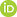Full Text:<1710>

Summary:<1367>

CLC number: TN47

On-line Access: 2017-12-04

Revision Accepted: 2016-12-20

Crosschecked: 2017-10-31

Cited: 0

Clicked: 5045

Citations:  Bibtex RefMan EndNote GB/T7714ORCID:

Mao-qun Yao

http://orcid.org/0000-0001-6484-4972

### -   Go to

Article info.
 Frontiers of Information Technology & Electronic Engineering  2017 Vol.18 No.10 P.1654-1664 http://doi.org/10.1631/FITEE.1601730Function synthesis algorithm based on RTD-based three-variable universal logic gates

 Author(s):  Mao-qun Yao, Kai Yang, Ji-zhong Shen, Cong-yuan Xu Affiliation(s):  Hangzhou Institute of Service Engineering, Hangzhou Normal University, Hangzhou 310036, China; more Corresponding email(s):   yaomaoqun@163.com Key Words:  Resonant tunneling device (RTD), Disjunctive decomposition algorithm, Universal logic gate, Truth value matrix, Function synthesis algorithm Share this article to： More <<< Previous Article|Next Article >>>

Mao-qun Yao, Kai Yang, Ji-zhong Shen, Cong-yuan Xu. Function synthesis algorithm based on RTD-based three-variable universal logic gates[J]. Frontiers of Information Technology & Electronic Engineering, 2017, 18(10): 1654-1664.

@article{title="Function synthesis algorithm based on RTD-based three-variable universal logic gates",
author="Mao-qun Yao, Kai Yang, Ji-zhong Shen, Cong-yuan Xu",
journal="Frontiers of Information Technology & Electronic Engineering",
volume="18",
number="10",
pages="1654-1664",
year="2017",
publisher="Zhejiang University Press & Springer",
doi="10.1631/FITEE.1601730"
}

%0 Journal Article
%T Function synthesis algorithm based on RTD-based three-variable universal logic gates
%A Mao-qun Yao
%A Kai Yang
%A Ji-zhong Shen
%A Cong-yuan Xu
%J Frontiers of Information Technology & Electronic Engineering
%V 18
%N 10
%P 1654-1664
%@ 2095-9184
%D 2017
%I Zhejiang University Press & Springer
%DOI 10.1631/FITEE.1601730

TY - JOUR
T1 - Function synthesis algorithm based on RTD-based three-variable universal logic gates
A1 - Mao-qun Yao
A1 - Kai Yang
A1 - Ji-zhong Shen
A1 - Cong-yuan Xu
J0 - Frontiers of Information Technology & Electronic Engineering
VL - 18
IS - 10
SP - 1654
EP - 1664
%@ 2095-9184
Y1 - 2017
PB - Zhejiang University Press & Springer
ER -
DOI - 10.1631/FITEE.1601730

Abstract:
Compared with complementary metal–oxide semiconductor (), the resonant tunneling device (RTD) has better performances; it is the most promising candidate for next-generation integrated circuit devices. The universal logic gate is an important unit circuit because of its powerful logic function, but there are few function synthesis algorithms that can implement an n-variable logical function by RTD-based universal logic gates. In this paper, we propose a new concept, i.e., the truth value matrix. With it a novel disjunctive decomposition algorithm can be used to decompose an arbitrary n-variable logical function into three-variable subset functions. On this basis, a novel function synthesis algorithm is proposed, which can implement arbitrary n-variable logical functions by RTD-based universal threshold logic gates (UTLGs), RTD-based three-variable XOR gates (XOR3s), and RTD-based three-variable universal logic gate (ULG3s). When this proposed function synthesis algorithm is used to implement an n-variable logical function, if the function is a directly disjunctive decomposition one, the circuit structure will be very simple, and if the function is a non-directly disjunctive decomposition one, the circuit structure will be simpler than when using only UTLGs or ULG3s. The proposed function synthesis algorithm is straightforward to program, and with this algorithm it is convenient to implement an arbitrary n-variable logical function by RTD-based universal logic gates.

### Reference

Altun, M., Riedel, M.D., 2012. Logic synthesis for switching lattices. IEEE Trans. Comput., 61(11):1588-1600.Beiu, V., Quintana, J.M., Avedillo, M.J., 2003. VLSI implementations of threshold logic—a comprehensive survey. IEEE Trans. Neur. Networks, 14(5):1217-1243.Bertacco, V., Damiani, M., 1997. The disjunctive decomposition of logic functions. IEEE/ACM Int. Conf. on Computer-Aided Design, p.78-82.Czajkowski, T.S., Brown, S.D., 2008. Functionally linear decomposition and synthesis of logic circuits for FPGAs. Comput.-Aided Des. Integr. Circ. Syst., 27(12):2236-2249.Falkowski, B.J., Kannurao, S., 2001. Analysis of disjoint decomposition of balanced Boolean functions through the Walsh spectrum. Comput. Dig. Techn., 148(2):71-78.Fan, D.L., Sharad, M., Roy, K., 2014. Design and synthesis of ultralow energy spin-memristor threshold logic. IEEE Trans. Nanotechnol., 13(3):574-583.Files, C.M., Perkowski, M.A., 2000. New multivalued functional decomposition algorithms based on MDDs. Comput.-Aided Des. Integr. Circ. Syst., 19(9):1081-1086.Hrynkiewicz, E., Kolodzinski, S., 2010. An Ashenhurst disjoint and non-disjoint decomposition of logic functions in Reed-Muller spectral domain. Proc. 17th Int. Conf. on Mixed Design of Integrated Circuits and Systems, p.200-204.

Iwai, H., 2013. Future of nano CMOS technology. Proc. Symp. on Microelectronics Technology and Devices, p.1-10.

Kolodzinski, S., Hrynkiewicz, E., 2009. An utilisation of Boolean differential calculus in variables partition calculation for decomposition of logic functions. 12th Int. Symp. on Design and Diagnostics of Electronic Circuits & Systems, p.34-37.Likharev, K.K., 2008. Hybrid CMOS/nanoelectronic circuits: opportunities and challenges. J. Nanoelectron. Opto-electron., 3(3):203-230.Liu, M.C., Lin, D.D., Pei, D.Y., 2011. Fast algebraic attacks and decomposition of symmetric Boolean functions. IEEE Trans. Inform. Theory, 57(7):4817-4821.Mazumder, P., Kulkarni, S., Bhattacharya, M., 1998. Digital circuit applications of resonant tunneling devices. Proc. IEEE, 86(4):664-686.Mirhoseini, S.M., Sharifi, M.J., Bahrepour, D., 2010. New RTD-based general threshold gate topologies and application to three-input XOR logic gates. J. Electr. Comput. Eng., 35(1):1-4.Muramatsu, N., Okazaki, H., Waho, T., 2005. A novel oscillation circuit using a resonate-tunneling diode. IEEE Int. Symp. on Circuits and Systems, p.2341-2344.Ngwira, S.M., Tshabalala, P., 2002. Neural network analysis for the identification of optimal variable orderings in the decomposition of complex logic functions. Comput. Dig. Techn., 149(5):240-244.Nikodem, M., 2013. Synthesis of multithreshold threshold gates based on negative differential resistance devices. IET Circ. Dev. Syst., 7(5):232-242.Wei, Y., Shen, J.Z., 2011. Novel universal threshold logic gate based on RTD and its application. Microelectron. J., 42: 851-854.Yao, M.Q., Yang, K., Xu, C.Y., et al., 2015. Design of a novel RTD-based three-variable universal logic gate. Front. Inform. Technol. Electron. Eng., 16(8):694-699.Zhang, R., Gupta, P., Zhong, L., 2005. Threshold network synthesis and optimization and its application to nanotechnologies. IEEE Trans. Comput.-Aided Des. Integr. Circ. Syst., 24(1):107-118.Zheng, Y.X., Huang, C., 2009. Complete logic functionality of reconfigurable RTD circuit elements. IEEE Trans. Nano-technol., 8(5):631-642.